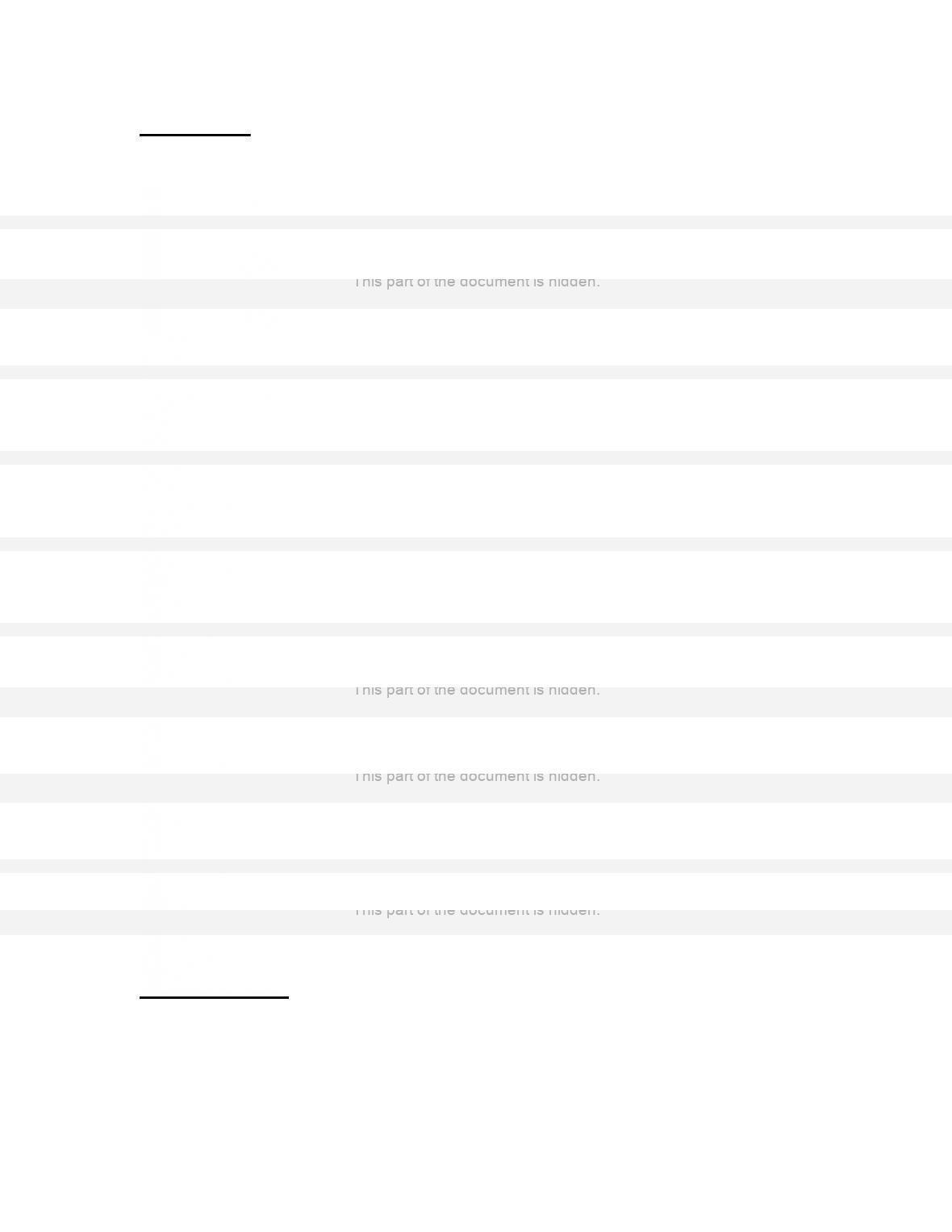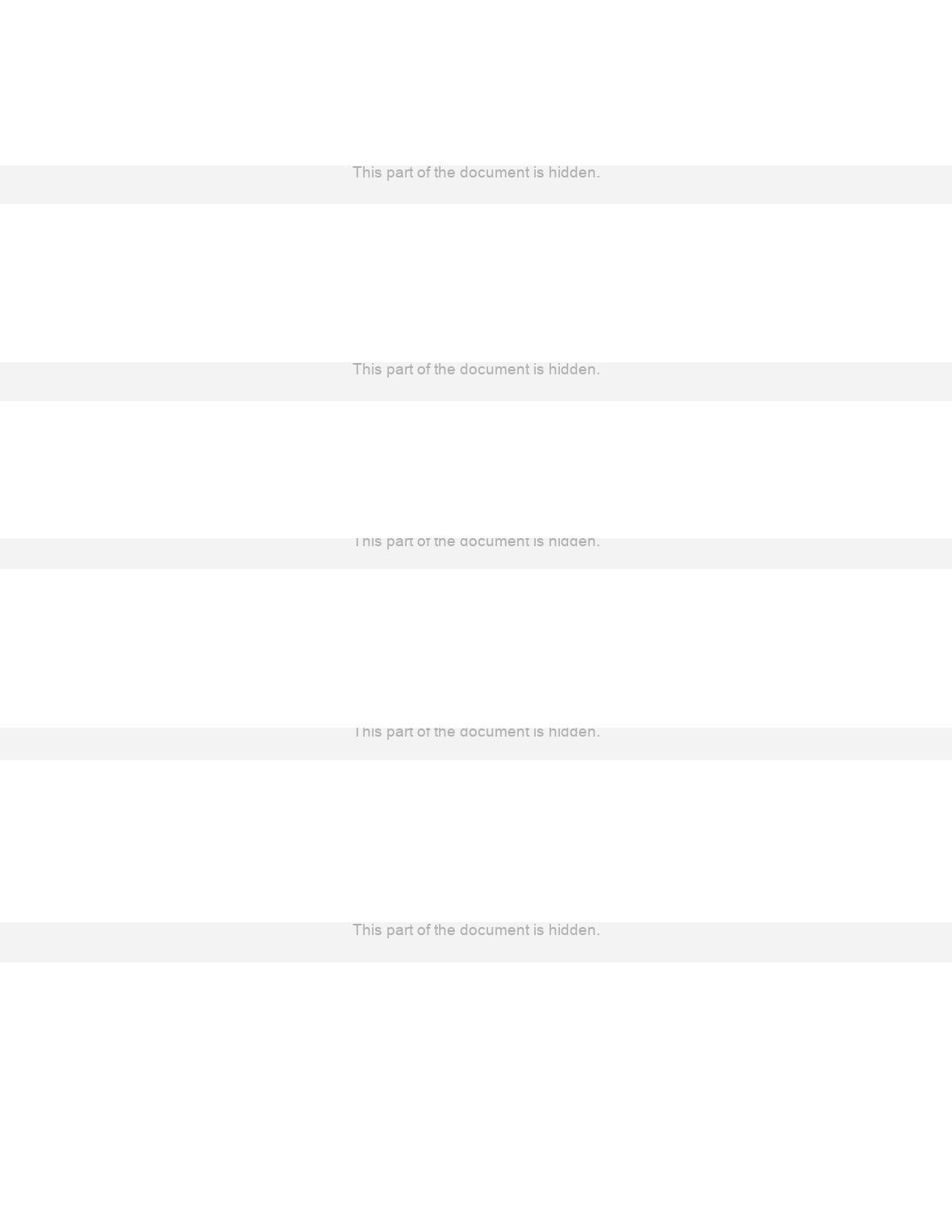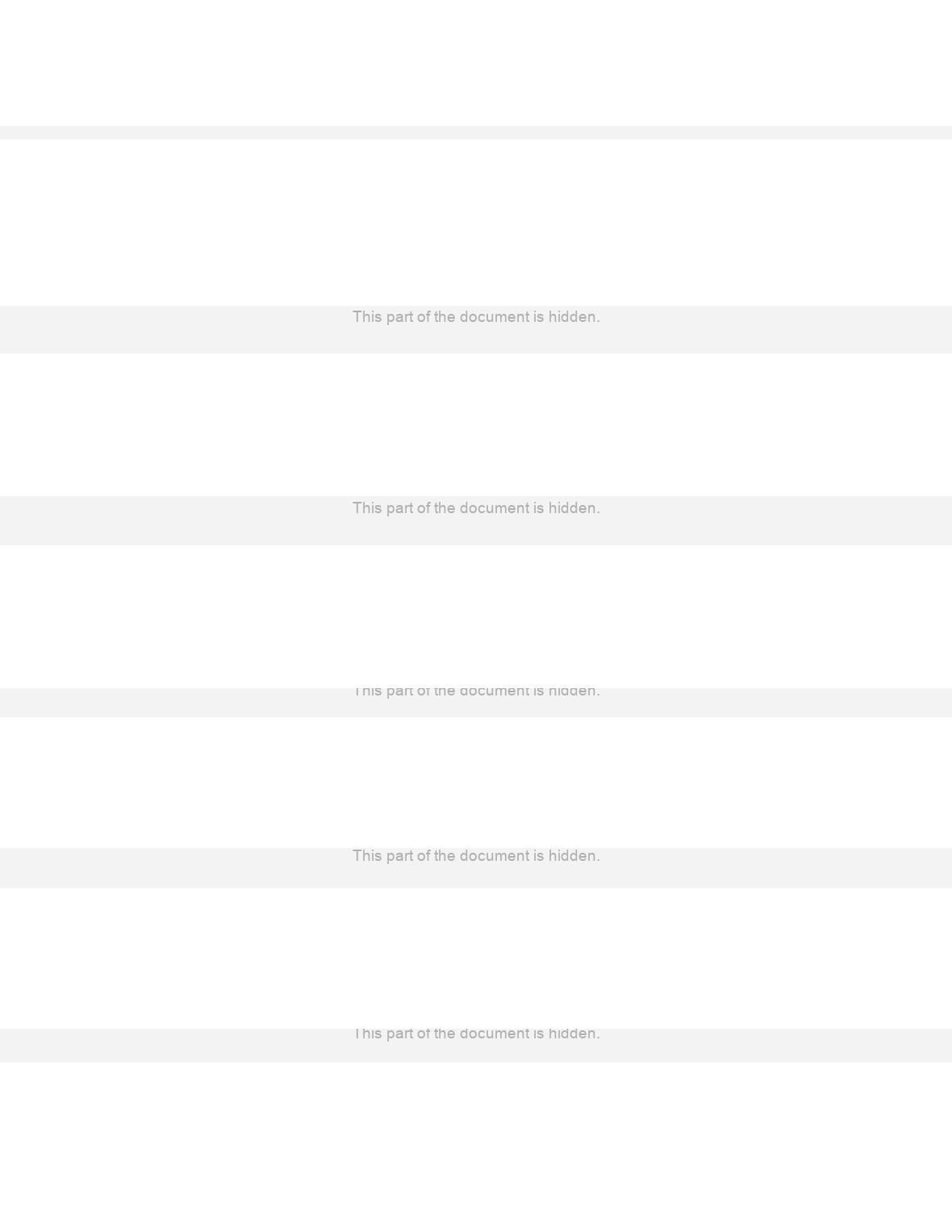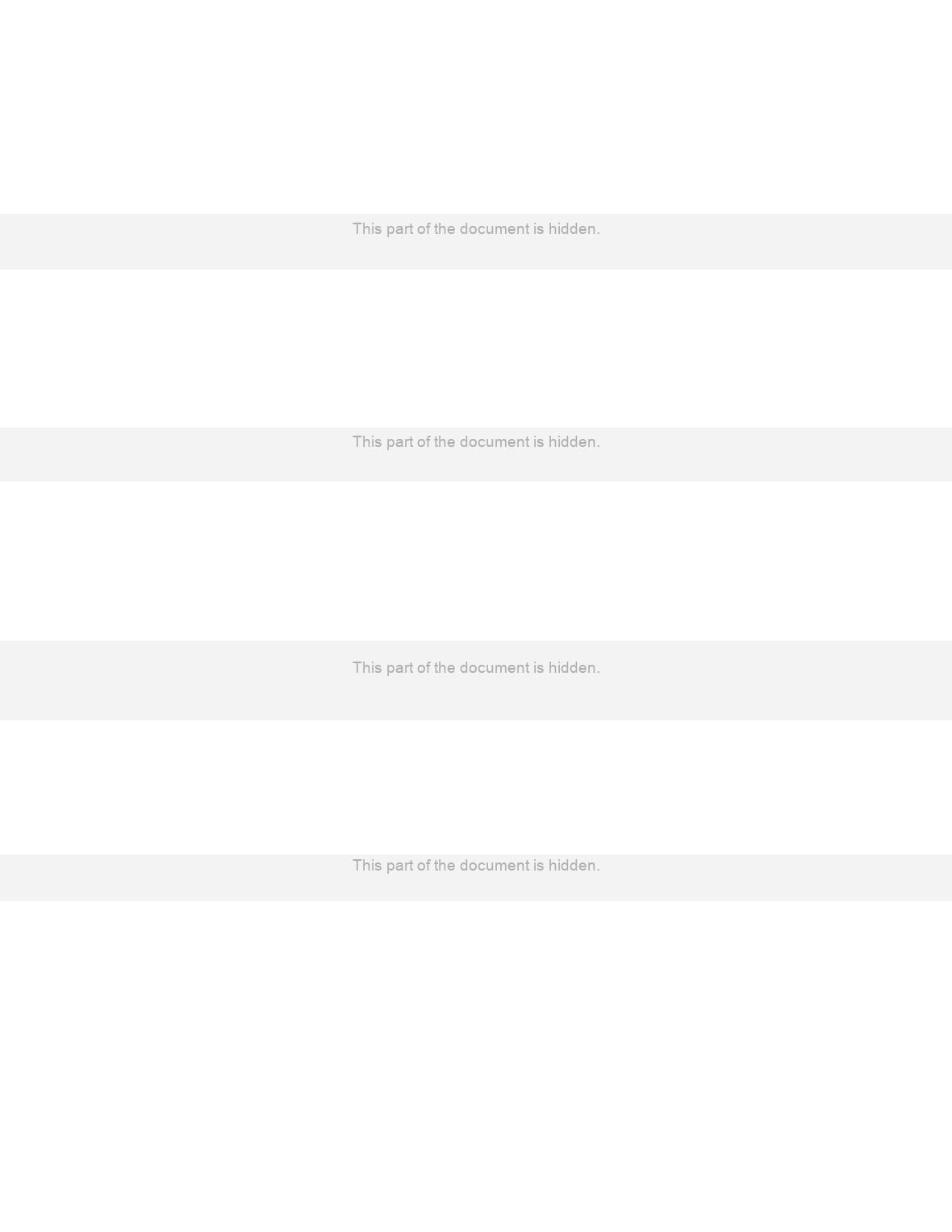Type
Quiz
Book Title
Computer Security Fundamentals (Pearson IT Cybersecurity Curriculum (ITCC)) 3rd Edition
ISBN 13
978-0789757463

### 978-0789757463 Chapter 8

February 27, 2021True / False
1. A virtual private network is a way to use the Internet to create a connection between a
remote user and a central location.
2. IPsec can only encrypt the packet data but not the header information.
3. L2TP uses IPsec for its encryption.
4. Kerberos is an authentication protocol that uses a ticket granting system that sends an
encrypted ticket to the user’s machine.
5. A digital signature is used to guarantee who sent a message. This is referred to as non-
repudiation.
6. Public key encryption is fast becoming the most widely used type of encryption
because there are no issues to deal with concerning distribution of keys.
7. PGP involves only private key encryption.
8. The most widely used symmetric key algorithm is Advanced Encryption Standard.
9. Cipher text is encrypted text.
10. Binary numbers are made up of 0s and 1s.
Multiple Choice
1. ______________ is the process to scramble a message or other information so that it
a. Encryption
b. Cryptographyc. Keying
d. Decryption
2. __________ is the art to write in or decipher secret code.
a. Encryption
b. Cryptography
c. Keying
d. Decryption
3. Which of these is NOT one of the two basic types of cryptography?
a. Asymmetric
b. Symmetric
c. Forward
d. None of the above
4. With asymmetric cryptography a different ______ is used to encrypt the message and
to decrypt the message.
a. Code
b. Key
c. Lock
d. Script
5. Using the __________ cipher you choose some number by which to shift each letter
of a text.
a. DC4
b. Caesar
c. Multi-alphabet substitution
d. ASCII
6. Using the _________ cipher you select multiple numbers by which to shift letters.
a. DC4
b. Caesar
c. Multi-alphabet substitution
d. ASCII7. Which of the following is an operation used on binary numbers not found in normal
math?
a. AND
b. PLUS
c. MINUS
d. None of the above
8. A(n) ________ refers to the bits that are combined with the plain text to encrypt it.
a. Key
b. Plain
c. Cipher text
d. Algorithm
9. ________ refers to unencrypted text.
a. Key
b. Plain text
c. Algorithm
d. Cipher text
10. __________refers to encrypted text.
a. Key
b. Plain text
c. Algorithm
d. Cipher text
11. A(n) ___________is a mathematical process for doing something.
a. Formula
b. Algorithm
c. Cipher
d. None of the above
12. Which of these is NOT a type of symmetric algorithm?a. Stream
b. Block
c. Transcription
d. None of the above
13. _________ is a block cipher that uses a variable-length key ranging from 32 to 448
bits.
a. 3DES
b. AES
c. Blowfish
d. RC4
14. With ______________ encryption, one key is used to encrypt a message, and another
is used to decrypt the message.
a. Private key
b. Public key
c. Cipher
d. None of the above
15. Windows stores passwords using a method called __________.
a. Hashing
b. Authentication
c. Kerberos
d. None of the above